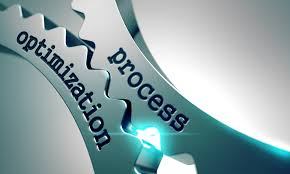# Process Optimization: An Enabler for Profit Maximization in Chemical, Oil and Gas IndustriesThis course will introduce the participants how to formulate optimization problems in our daily processes, to know which solution techniques to use, and to be able to use simple yet powerful approach/method/software to optimize our processes. The course covers steady-state optimization which is applied broadly ranging from molecular scale to unit operations (e.g. process design and engineering), plant sites (e.g. resources minimization), and until enterprise level (e.g. supply chain management).

The objective of the training/course are as follows:

• To introduce the skill to develop mathematical models and to formulate optimization problems in industrial processes.
• To demonstrate systematic problem solving involving Linear Programming, Integer Programming, and Non-Linear Programming, using analytical techniques and computational tools.

Course content of the training:

• Overview and introduction of optimization in daily life and industrial examples
• needs of optimization
• industrial and daily life examples
• Principles of models building and introduction to optimization
• Framework, conservation laws, constitutive relations, empirical models, model simplifications
• Objective functions
• Variables and constraints
• Linear Programming (LP)
• Formulation
• Graphical solution
• Simplex method
• Excel solver demonstration
• Integer Programming (IP)
• Integer and binary variables
• Branch and bound
• Excel solver demonstration
• Non-Linear Programing (NLP)
• Lagrange function
• Newton’s method